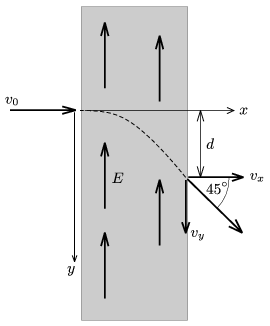Mathematical and Physical Journal
for High Schools
Issued by the MATFUND Foundation
 Already signed up? New to KöMaL?

#Problem P. 4357. (May 2011)

P. 4357. An electron enters to a region of uniform electric field, which is perpendicular to its velocity. The speed of the electron is 60% of the speed of light. When it leaves the region where there is electric field the angle between its original and final velocity is 45o. a) What is the speed of the electron when it leaves the region of the electric field? b) What is the displacement d of the electron, if the electric field strength is E=510 kV/m? (The rest energy of the electron is m0c2=510 keV.)(5 pont)

Deadline expired on June 10, 2011.

Sorry, the solution is available only in Hungarian. Google translation

Megoldás. $\displaystyle a)$ A fénysebesség 72,8 százalékával.

$\displaystyle b)$ $\displaystyle d=$20,8 cm.

### Statistics:

 49 students sent a solution. 5 points: Antalicz Balázs, Barta Szilveszter Marcell, Béres Bertold, Bolgár Dániel, Czigány Máté Gábor, Fülep Andrea , Horicsányi Attila, Jéhn Zoltán, Jenei Márk, Juhász Péter, Maknics András, Nagy 111 Miklós, Nagy Zsolt, Pataki Bálint Ármin, Pázmán Koppány, Pető János, Szabó 928 Attila, Szigeti Bertalan György, Várnai Péter, Vuchetich Bálint. 4 points: Dinev Georgi, Filep Gábor, Szélig Áron. 3 points: 1 student. 2 points: 12 students. 1 point: 5 students. 0 point: 8 students.

Problems in Physics of KöMaL, May 2011